Courses

# Previous Year Questions: Laws of Motion

## 9 Questions MCQ Test Physics For JEE | Previous Year Questions: Laws of Motion

Description
This mock test of Previous Year Questions: Laws of Motion for JEE helps you for every JEE entrance exam. This contains 9 Multiple Choice Questions for JEE Previous Year Questions: Laws of Motion (mcq) to study with solutions a complete question bank. The solved questions answers in this Previous Year Questions: Laws of Motion quiz give you a good mix of easy questions and tough questions. JEE students definitely take this Previous Year Questions: Laws of Motion exercise for a better result in the exam. You can find other Previous Year Questions: Laws of Motion extra questions, long questions & short questions for JEE on EduRev as well by searching above.
QUESTION: 1

### Mass of the object is quantitative measure of its inertia stated law is newton's

Solution:

Mass of the object is quantitative measure of its inertia stated law is Newton's First Law.

QUESTION: 2

### Two blocks A and B masses 2m and m, respectively, are connected by a massless and inextensible string. The whole system is suspended by a massless spring as shown in the figure. The magnitudes of acceleration of A and B, immediately after the string is cut, are respectively.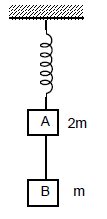[JEE 2006]

Solution:

Before the string is cut the force applied by the spring is 3mg and tension in string is mg
Once the spring is cut, the tension comes to zero suddenly,
Thus acceleration of block b gets g downwards,
Whereas acceleration of a becomes g/2 upwards because the force from the spring is still the same at the moment the string is cut.

QUESTION: 3

### A circular disc with a groove along its diameter is placed horizontally. A block of mass 1 kg is placed as shown. The co-efficient of friction between the block and all surfaces of groove in contact is m = 2/5. The disc has an acceleration of 25 m/s2. Find the acceleration of the block with respect to disc.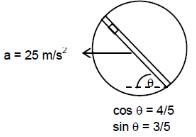[JEE 2006]

Solution: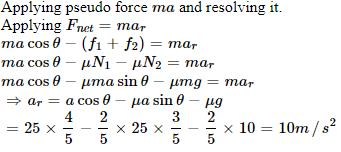QUESTION: 4

STATEMENT-1 A cloth Covers a table. Some dishes are kept on it. The cloth can be pulled out without dislodging the dishes from the table

because

STATEMENT-2 For every action there is an equal and opposite reaction

Solution:

“A cloth covers a table. Some dishes are kept on it. The cloth can be pulled out without dislodging the dishes from the table.” This is a very common phenomenon and can be seen when the cloth is pulled very quickly. But it can’t be explained by the 3rd Law of Motion.

QUESTION: 5

Statement-1 It is easier to pull a heavy object than to push it on a level ground.

and

Statement-2 The magnitude of frictional force depends on the nature of the two surfaces in contact .

[ JEE 2008]

Solution:

Both statements are independently true but 2 is not the correct explanation of 1.
The reason for statement 1 is that while pulling, a component of force reduces the normal reaction and hence reduces the friction force.

QUESTION: 6

A block of base 10 cm × 10 cm and height 15 cm is kept on an inclined plane. The coefficient of friction between them is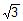. The inclination q of this inclined plane from the horizontal plane is gradually increased from 0º. Then                                                                                                 [jee 2009]

Solution: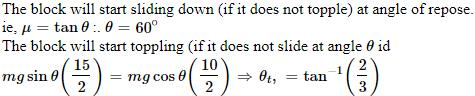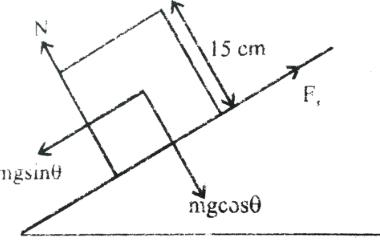QUESTION: 7

A block of mass m is on an inclined plane of angle q. The coefficient of friction betwen the block and the plane is m and tan q > m. The block is held stationary by applying a force P parallel to the plane. The direction of force pointing up the plane is taken to the positive. As P is varied from P = mg (sin q _ m cos q ) to Pz = mg (sin q + m cos q), the frictional force f versus P graph will look like              [jee 2010]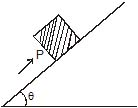Solution:

f= sin Q−P

arry lineary
fi=mg sinθ−mg(sinθ − Hcosθ)
Hmg cosθ
1​=mgsinθ−mg(sinθ + Hcosθ)f
=−Hnmg sinθ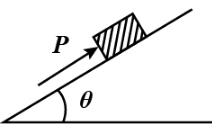QUESTION: 8

A block is moving on an inclined plane making an angle 45º with horizontal and the coefficient of friciton is μ. the force required to just push it up the inclined plane is 3 times the force requried to just prevent it from sliding down. If we define N = 10μ, then N is

[jee 2011]

Solution: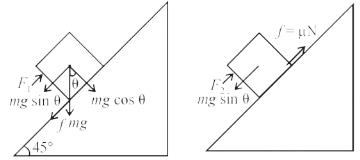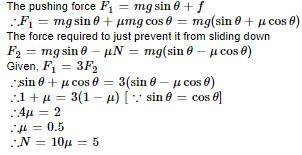*Multiple options can be correct
QUESTION: 9

A small block of mass of 0.1 kg lies on a fixed inclined plane PQ which makes an angle with the horizontal. A horizontal force of 1 N acts on the block through its center of mass as shown in the figure. The block remains stationary if (take g = 10 m/s2)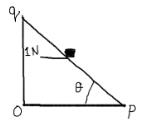Solution: